# Basic Vector Operations

Both a magnitude and a direction must be specified for a vector quantity, in contrast to a scalar quantity which can be quantified with just a number. Any number of vector quantities of the same type (i.e., same units) can be combined by basic vector operations.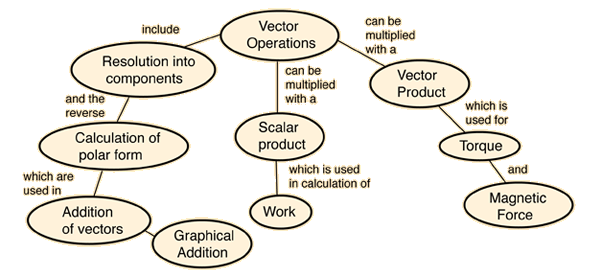Caution! This is a large HTML document. You need to wait for it to load completely in order for all the links above to operate.
Index

Math of vectors

 HyperPhysics***** Mechanics R Nave
Go BackAdding two vectors A and B graphically can be visualized like two successive walks, with the vector sum being the vector distance from the beginning to the end point. Representing the vectors by arrows drawn to scale, the beginning of vector B is placed at the end of vector A. The vector sum R can be drawn as the vector from the beginning to the end point. The process can be done mathematically by finding the components of A and B, combining to form the components of R, and then converting to polar form.
Index

Vector concepts

 HyperPhysics***** Mechanics R Nave
Go Back

# Example of Vector Components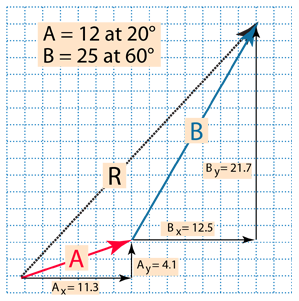Finding the components of vectors for vector addition involves forming a right triangle from each vector and using the standard triangle trigonometry.The vector sum can be found by combining these components and converting to polar form.
Index

Vector concepts

 HyperPhysics***** Mechanics R Nave
Go Back

# Polar Form ExampleAfter finding the components for the vectors A and B, and combining them to find the components of the resultant vector R, the result can be put in polar form bySome caution should be exercised in evaluating the angle with a calculator because of ambiguities in the arctangent on calculators.
Index

Vector concepts

 HyperPhysics***** Mechanics R Nave
Go Back

# Combining Vector Components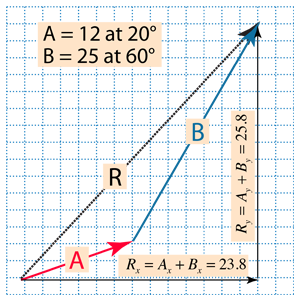After finding the components for the vectors A and B, these components may be just simply added to find the components of the resultant vector R.The components fully specify the resultant of the vector addition, but it is often desirable to put the resultant in polar form.
Index

Vector concepts

 HyperPhysics***** Mechanics R Nave
Go Back

# Resolving a Vector Into Components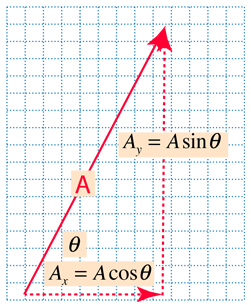Vectors are resolved into components by use of the triangle trig relationships. You may change the length or angle of the polar form of the vector, and the components will be calculated below. For vector A= at angle degrees, the horizontal component is = and the vertical component is = The input to the boxes for units is arbitrary; they serve to emphasize that the process of vector addition is independent of the units of the vector.
Note: this Javascript routine does not work for angle exactly equal to 90° .

Index

Vector concepts

 HyperPhysics***** Mechanics R Nave
Go Back

# Magnitude and Direction from Components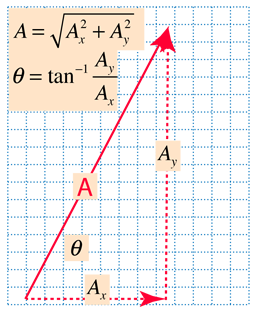If the components of a vector are known, then its magnitude and direction can be calculated with the use of the Pythagorean relationship and triangle trig. This is called the polar form of the vector. If the horizontal component is = and the vertical component is = , then the magnitude is = and the angle is = degrees. The input to the boxes for units is arbitrary; they serve to emphasize that the process of vector addition is independent of the units of the vector. Some caution should be exercised in evaluating the angle with a calculator because of ambiguities in the arctangent on calculators.
Index

Vector concepts

 HyperPhysics***** Mechanics R Nave
Go Back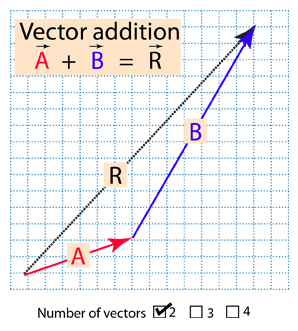Vector addition involves finding vector components, adding them and finding the polar form of the resultant. The addition of vector A= at degrees, and vector B= at degrees, yields components:+ =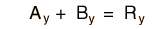+ = The resultant has magnitude R = and angle = degrees.
Note: There are some combinations of angles and values, like those where an exactly zero result is anticipated or an angle of exactly 90 degrees, where the Javascript routine is unstable and gives erroneous values. Usually you can try values very close to such conditions to test the accuracy.
Index

Vector concepts

 HyperPhysics***** Mechanics R Nave
Go Back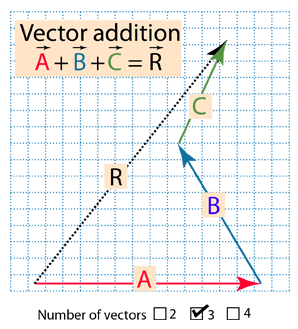Vector addition involves finding vector components, adding them and finding the polar form of the resultant. The addition of vectorsA= at degrees, B= at degrees,and C= at degrees yields components:+ + =+ + = The resultant has magnitude R = and angle = degrees.
Index

Vector concepts

 HyperPhysics***** Mechanics R Nave
Go Back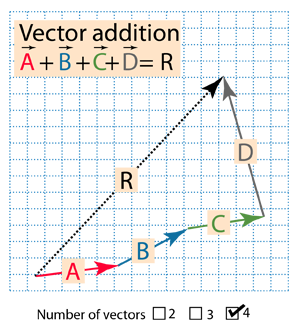Vector addition involves finding vector components, adding them and finding the polar form of the resultant. The addition of vectorsA= at degrees, B= at degrees, C= at degrees, D= at degrees, yields components: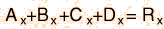+++=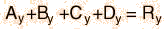+++= The resultant has magnitude R = and angle = degrees.